﻿ 融资约束、人工智能与经济增长《财经研究》
2022第48卷第8期

1. 首都经济贸易大学 经济学院，北京 100070;
2. 中国人民大学 劳动人事学院，北京 100872

Financial Constraints, Artificial Intelligence, and Economic Growth
Zhang Xiaomeng1, Liu Xiangbo2
1. School of Economics, Capital University of Economics and Business, Beijing 100070, China;
2. School of Labor and Human Resources, Renmin University of China, Beijing 100872, China
Summary: Intelligent manufacturing is considered to be a top priority for promoting manufacturing industry transformation. We must stay the course of AI technology application and develop intelligent manufacturing, in order to achieve the strategic goal of becoming a manufacturing power and grasp the opportunity of building new advantages in international competition. This paper constructs a task-based model that includes financing constraints, endogenous AI technological innovation and labor market frictions, and discusses the impact of financing constraints on the level of intelligent manufacturing, and thus how output and employment levels are affected. This paper finds that: First, due to the heterogeneity in the possibilities of being replaced by AI for different tasks, easing the financing constraints of firms engaged in AI technological innovation can increase the proportion of automated tasks, and promote the use of automation capital. Second, the development of AI has a heterogeneous impact on the labor market of different skills through the negative displacement effect and the positive creation effect. Specifically, on the one hand, the application of AI technology leads to the replacement of labor with automation capital, increasing the unemployment rate. On the other hand, the development of intelligent manufacturing increases the expected profit and enhances the incentives for firms to enter the market, thereby promoting output growth and reducing the unemployment rate. Overall, intelligent manufacturing promotes the aggregate output and decreases the unemployment rate. Consequently, we suggest that the role of the financial market should be emphasized in the process of intelligent manufacturing transformation, and the relationship between automation and employment should be coordinated to reduce the negative effect of automation on the labor market. Feasible strategies include encouraging market entry, promoting industrial integration, eliminating labor market barriers, and making the skill structure of labor force adapt to the transformation and upgrading of intelligent manufacturing. The contributions are as follows: First, the existing literature mainly focuses on the impact of AI but pays little attention to the endogenous progress of AI technology. We argue that the level of intelligent manufacturing is determined by AI technological innovation, and establish a theoretical model that includes endogenous AI technological innovation. Second, the existing literature on AI rarely considers the labor market or financial market frictions. We introduce the labor market search and matching frictions and financial constraints on R&D investment into the task-based model framework, to provide a credible theoretical framework for further research. Third, we analyze the impact of easing financial constraints on improving intelligent manufacturing and promoting economic growth, and propose policies that can mitigate or even reverse the negative displacement effect of automation on the labor market.
Key words: financial constraints    artificial intelligence    technological innovation    economic growth    employment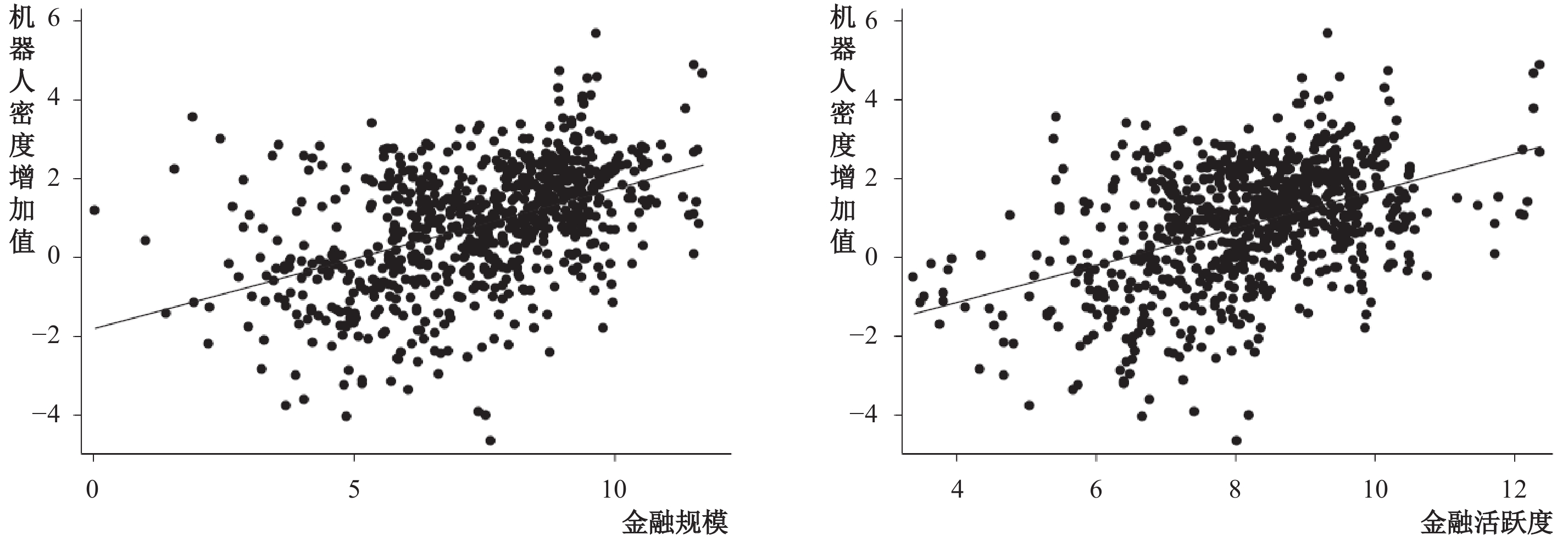图 1 金融规模、金融活跃度与智能化生产水平 注：本文参考Acemoglu和Restrepo（2020），使用工业机器人保有量与制造业部门就业人数之比，即工业机器人密度（robot density）衡量智能化生产水平。工业机器人保有量数据来源为国际机器人联合会（IFR）数据库，制造业部门就业人数来自联合国工业发展组织（UNIDO）数据库，并对各年度机器人密度增加值取对数。遵循Beck和Levine（2002）的构造方法，使用金融中介对私人部门信贷余额和GDP之比与股票市场市值占GDP比重的对数之和度量金融规模，使用金融中介对私人部门信贷余额和GDP之比与股票市场交易额占GDP比重的对数之和衡量金融活跃度。样本包括53个国家和地区，时间跨度为1993年至2017年。

1. 模型设定

 $Y = {\left( {\int_0^1 {Y_i^{\frac{{\varepsilon - 1}}{\varepsilon }}di} } \right)^{\frac{\varepsilon }{{\varepsilon - 1}}}},\varepsilon > 0$ (1)

 ${y_{N,i}} = {\left( {{Y_{N,i}}{\text{/}}Y} \right)^{ - 1{\text{/}}\varepsilon }},{y_{R,i}} = {\left( {{Y_{R,i}}{\text{/}}Y} \right)^{ - 1{\text{/}}\varepsilon }}$ (2)

（1）无法使用人工智能替代的任务 $i \in (\alpha ,1)$ 。假设 ${\Pi _{N,i}}$ 表示在无法使用人工智能生产的任务中匹配岗位 ${N_i}$ 的价值， ${V_{N,i}}$ 表示空置岗位的价值， ${w_{N,i}}$ 为工资水平， ${s_L}$ 为劳动力离职概率， $r$ 为贴现因子。 ${\Pi _{N,i}}$ 可以表示为：

 $r{\Pi _{N,i}} = {y_{N,i}} - {w_{N,i}} - {s_L}\left( {{\Pi _{N,i}} - {V_{N,i}}} \right)$ (3)

 $r{V_{N,i}} = - {c_{N,i}} + {q_{N,i}}\left( {{\Pi _{N,i}} - {V_{N,i}}} \right)$ (4)

 $r{E_{N,i}} = {w_{N,i}} - {s_L}\left( {{E_{N,i}} - {U_{N,i}}} \right)$ (5)
 $r{U_{N,i}} = {m_{N,i}}\left( {{E_{N,i}} - {U_{N,i}}} \right)$ (6)

（2）可能实现人工智能替代劳动力生产的任务 $i \in (0,\alpha )$ 。对此类任务而言，企业可以选择投入固定的研发成本，以一定概率实现智能化生产，也可以选择不进行人工智能技术研发，继续使用劳动力进行生产。如果企业选择不进行人工智能技术研发，则该任务与任务 $i \in (\alpha ,1)$ 相同，仅能够使用劳动力生产，岗位状态为 $N$ ，在此不进行重复说明。如果企业选择进行人工智能技术研发，则有一定概率实现智能化生产。我们用 ${\phi _i}$ 表示研发成本， ${\mu _i}$ 表示实现智能化生产的概率。根据上文设定，指数 $i$ 表示生产任务实现人工智能技术替代的难易程度，指数 $i$ 越大，研发成功从而实现智能化生产的概率 ${\mu _i}$ 越小，即 $\mu _i^{'} < 0$ 。假设 ${\Pi _{R,i}}$ 表示选择进行人工智能技术研发的匹配岗位 ${R_i}$ 的价值， ${V_{R,i}}$ 表示空置岗位的价值， ${w_{R,i}}$ 表示该岗位的工资水平，假设实现智能化生产后，岗位状态由 $R$ 变为 $A$ ${\Pi _{A,i}}$ 表示智能化生产岗位的价值，可知：

 $r{\Pi _{R,i}} = {y_{R,i}} - {w_{R,i}} - {\phi _i} - {s_L}\left( {{\Pi _{R,i}} - {V_{R,i}}} \right) + {\mu _i}\left( {{\Pi _{A,i}} - {\Pi _{R,i}}} \right)$ (7)
 $r{\Pi _{A,i}} = \rho {y_{R,i}} - z - {s_A}\left( {{\Pi _{A,i}} - {V_{R,i}}} \right)$ (8)

 $r{V_{R,i}} = - {c_{R,i}} + {q_{R,i}}\left( {{\Pi _{R,i}} - {V_{R,i}}} \right)$ (9)

 $r{E_{R,i}} = {w_{R,i}} - \left( {{s_L} + {\mu _i}} \right)\left( {{E_{R,i}} - {U_{R,i}}} \right)$ (10)
 $r{U_{R,i}} = {m_{R,i}}\left( {{E_{R,i}} - {U_{R,i}}} \right)$ (11)

 ${\Pi _{R,i}} \geqslant {\Pi _{N,i}}$ (12)

 ${c_{\kappa ,i}} = {q_{\kappa ,i}}{\Pi _{\kappa ,i}}$ (13)

 $\mathop {\max }\limits_{{w_{\kappa ,i}}} {\mkern 1mu} {\left( {{E_{\kappa ,i}} - {U_{\kappa ,i}}} \right)^\beta }{\left( {{\Pi _{\kappa ,i}} - {V_{\kappa ,i}}} \right)^{1 - \beta }}$ (14)

 ${w_{N,i}} = \frac{{\beta \left( {r + {s_L} + {m_{N,i}}} \right){y_{N,i}}}}{{r + {s_L} + \beta {m_{N,i}}}}$ (15)
 ${w_{R,i}} = \frac{{\beta \left( {r + {s_L} + {m_{R,i}} + {\mu _i}} \right)\left( {{y_{R,i}} - {\phi _i} + {\mu _i}{\Pi _{A,i}}} \right)}}{{r + {s_L} + {\mu _i} + \beta {m_{R,i}}}}$ (16)

 ${\Pi _{N,i}} = \frac{{(1 - \beta ){y_{N,i}}}}{{r + {s_L} + \beta {m_{N,i}}}}$ (17)
 ${\Pi _{R,i}} = \frac{{(1 - \beta )({y_{R,i}} - {\phi _i} + {\mu _i}{\Pi _{A,i}})}}{{r + {s_L} + {\mu _i} + \beta {m_{R,i}}}}$ (18)

 ${\Pi _{A,i}} = \frac{{\rho {y_{R,i}} - z}}{{r + {s_A}}}$ (19)

2. 基准模型的稳态

 ${e_{N,i}} = \frac{{{m_{N,i}}{L_i}}}{{{s_L} + {m_{N,i}}}},{\text{ }}{u_{N,i}} = \frac{{{s_L}{L_i}}}{{{s_L} + {m_{N,i}}}},{\text{ }}{e_{R,i}} = \frac{{{m_{R,i}}{L_i}}}{{{s_L} + {\mu _i} + {m_{R,i}}}},{\text{ }}{u_{R,i}} = \frac{{\left( {{s_L} + {\mu _i}} \right){L_i}}}{{{s_L} + {\mu _i} + {m_{R,i}}}}$ (20)

 ${e_{A,i}} = \frac{{{\mu _i}{e_{R,i}}}}{{{s_A}}}$ (21)

 $\frac{{{c_{N,i}}}}{{{q_{N,i}}}} = \frac{{(1 - \beta ){y_{N,i}}}}{{r + {s_L} + \beta {m_{N,i}}}}$ (22)
 $\frac{{{c_{R,i}}}}{{{q_{R,i}}}} = \frac{{(1 - \beta )({y_{R,i}} - {\phi _i} + {\mu _i}{\Pi _{A,i}})}}{{r + {s_L} + {\mu _i} + \beta {m_{R,i}}}}$ (23)

 $\frac{{{y_{R,i}} - {\phi _i} + {\mu _i}{\Pi _{A,i}}}}{{r + {s_L} + {\mu _i} + \beta {m_{R,i}}}} \geqslant \frac{{{y_{N,i}}}}{{r + {s_L} + \beta {m_{N,i}}}}$ (24)

 ${\mu ^*} = {\mu _{{\alpha ^*}}} = \frac{{{y_{N,{\alpha ^*}}} - \left( {{y_{R,{\alpha ^*}}} - {\phi _{{\alpha ^*}}}} \right)}}{{{\Pi _{A,{\alpha ^*}}} - {{\left( {1 - \beta } \right)}^{ - 1}}{\Pi _{N,{\alpha ^*}}}}}$ (25)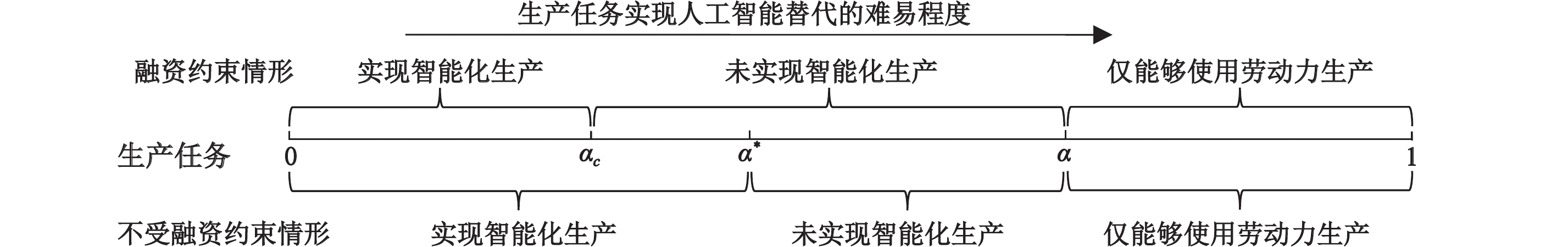图 2 企业人工智能研发决策和融资约束的影响 注：图2下半部分表示基准模型中企业的人工智能研发决策。其中，不同生产任务按照人工智能替代劳动难度递增排列，$\alpha^{*}$表示进行人工智能技术研发的任务阈值。上半部分表示受到融资约束情形时企业的人工智能研发决策，$\alpha_{c}$表示该情形下进行人工智能技术研发的任务阈值。

 $\frac{u}{L} = \dfrac{{\int_0^{\alpha *} {{u_{R,i}}di + \int_{\alpha *}^1 {{u_{N,i}}di} } }}{{\int_0^1 {{L_i}di} }}$ (26)

 ${\phi _i} \leqslant \gamma {\Pi _{R,i}}$ (27)

（1）如果 $\gamma$ 高于临界值 $\gamma ^{*}=\phi_{i} / \Pi_{N{,\alpha *}}$ ，即对给定的研发成本 ${\phi _i}$ ，满足 $\phi_{i} < \gamma \Pi_{N,\alpha *}$ 。这时，基准模型中选择进行人工智能技术研发的企业，其匹配岗位价值一定满足 ${\phi _i} < \gamma {\Pi _{R,i}}$ 。因此，融资约束不会影响企业研发决策。在均衡条件下，智能化生产任务占比仍为 $\alpha^{*}$ ，融资约束不会影响智能化生产水平。

（2）如果 $\gamma$ 低于临界值 ${\gamma ^*} = {\phi _i}/{\Pi _{N,{\alpha ^*}}}$ ，则基准模型中选择进行人工智能技术研发的企业可能受到融资约束。我们将融资约束（27）式代入（18）式可以证明，当且仅当研发成功概率高于一定临界值 ${\mu _c}$ 时，企业才能够进行人工智能技术研发：

 ${\mu _i} \geqslant {\mu _c} = \frac{{{\phi _{{\alpha _c}}}\left( {r + {s_L} + \beta {m_{R,{\alpha _c}}}} \right) - \gamma \left( {1 - \beta } \right)\left( {{y_{R,{\alpha _c}}} - {\phi _{{\alpha _c}}}} \right)}}{{\gamma \left( {1 - \beta } \right){\Pi _{A,{\alpha _c}}} - {\phi _{{\alpha _c}}}}}$ (28)

 ${\mu _c} > \frac{{{y_{N,{\alpha ^*}}} - \left( {{y_{R,{\alpha ^*}}} - {\phi _{{\alpha ^*}}}} \right)}}{{{\Pi _{A,{\alpha ^*}}} - {{\left( {1 - \beta } \right)}^{ - 1}}{\Pi _{N,{\alpha ^*}}}}} = {\mu ^*}$ (29)

 $\frac{{\partial {\mu _c}}}{{\partial \gamma }} < 0,{\text{ }}\frac{{\partial {\alpha _c}}}{{\partial \gamma }} > 0$ (30)

 ${e_{N,i}} = \frac{{{m_{N,i}}{L_i}}}{{{s_L} + {m_{N,i}}}},{\text{ }}{e_{R,i}} = \frac{{{m_{R,i}}{L_i}}}{{{s_L} + {\mu _i} + {m_{R,i}}}},{\text{ }}{e_{A,i}} = \frac{{{\mu _i}{e_{R,i}}}}{{{s_A}}}$ (31)

 ${Y_{N,i}} = {e_{N,i}},{\text{ }}{Y_{R,i}} = {e_{R,i}} + \rho {e_{A,i}},{\text{ }}Y = {\left( {\int_0^{{\alpha _c}} {Y_{R,i}^{\frac{{\varepsilon - 1}}{\varepsilon }}di + } \int_{{\alpha _c}}^1 {Y_{N,i}^{\frac{{\varepsilon - 1}}{\varepsilon }}di} } \right)^{\frac{\varepsilon }{{\varepsilon - 1}}}}$ (32)

1. 参数赋值

 参数赋值 参考文献 贴现率 $r = 0.004$ 月度贴现率 匹配函数参数 $\sigma = 0.5$ ，工人谈判势力 $\beta = 0.5$ Battisti等（2018） 智能资本相对生产率 $\rho = 3$ Acemoglu和Restrepo（2020） 替代弹性 $\varepsilon = {\text{2}}$ Oberfield和Raval（2021） 离职率 ${s_L} = 0.022$ 张晓娣（2016） 智能资本折旧率 ${s_A} = 0.007$ ，智能资本租金 $z = 0.011$ International Federation of Robotics（2019） 具备人工智能技术替代可能性的生产任务占比 $\alpha = 0.{\text{46}}$ Nedelkoska和Quintini（2018） 参数校准 参数说明 空置岗位成本 ${c_{N,i}} = {c_{R,i}} = 0.641$ 稳态失业率 匹配效率参数 ${\psi _{N,i}} = {\psi _{R,i}} = 0.403$ 稳态劳动力市场紧度 研发效率参数 $\bar \mu = 41$ 基准模型下实现智能化生产的任务占比 研发成本参数 $\phi = 0.005$ 基准模型下的智能资本密度 融资约束参数 $\gamma = 0.0017$ 融资约束情形下的智能资本密度

2. 定量结果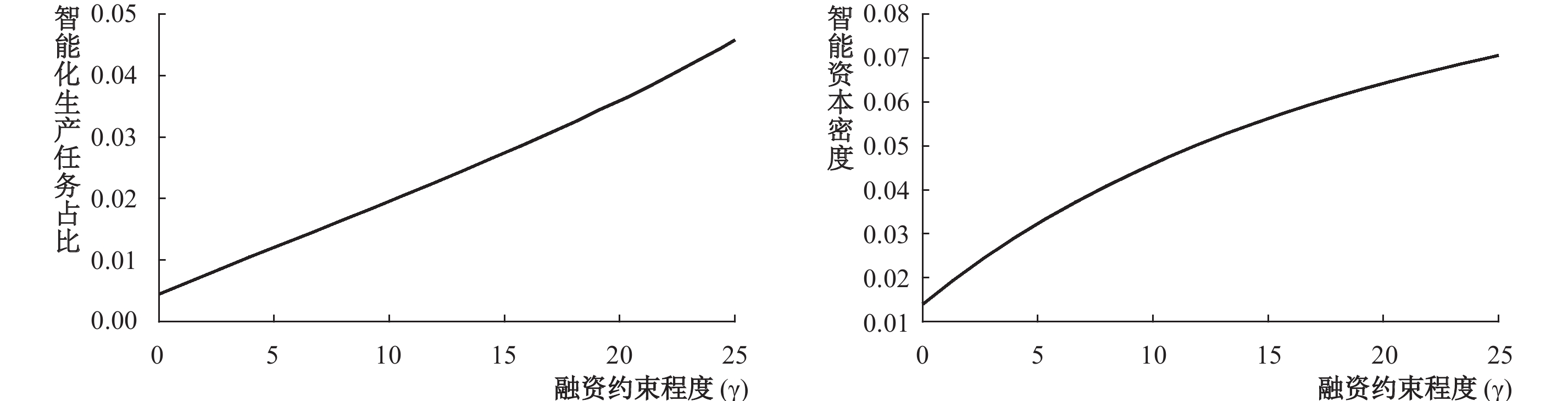图 3a 融资约束对实现智能化生产的任务占比和智能资本密度的影响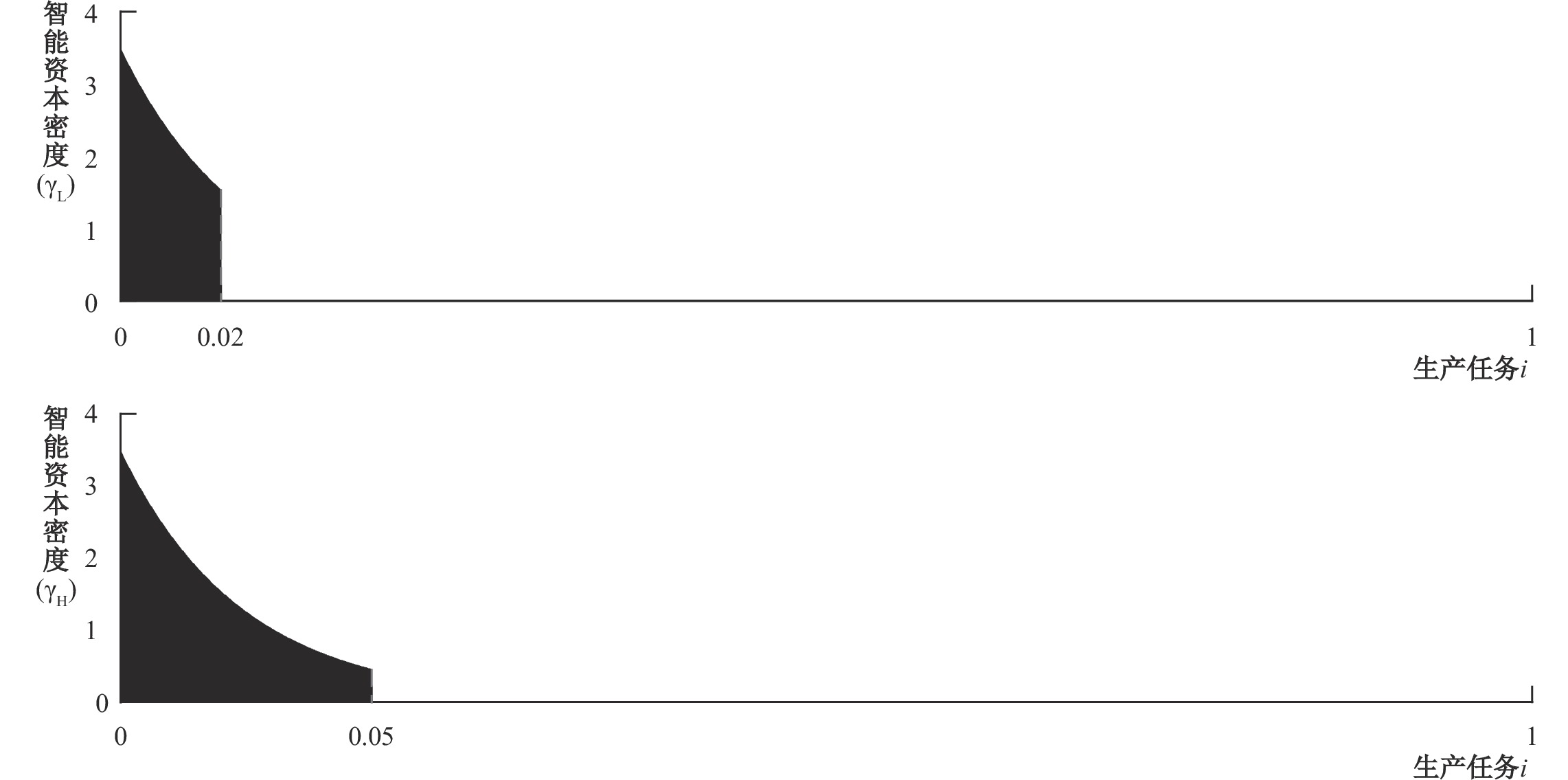图 3b 放松融资约束对智能化生产任务阈值和各生产任务智能资本密度的影响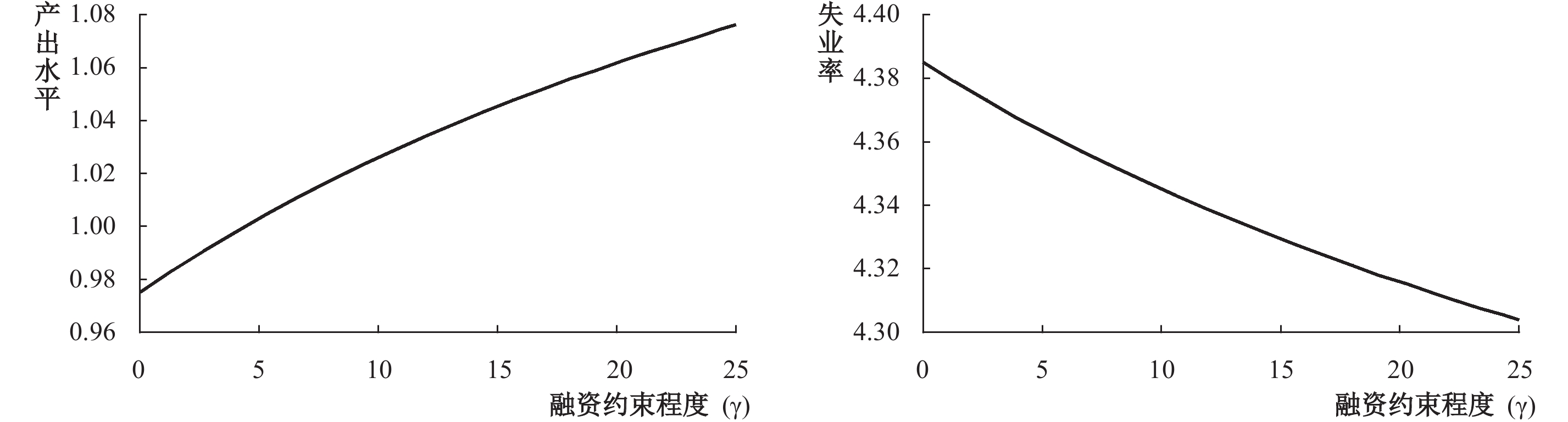图 4a 智能化生产水平提高对产出水平和失业率的影响

3. 机制检验图 4b 放松融资约束对就业的影响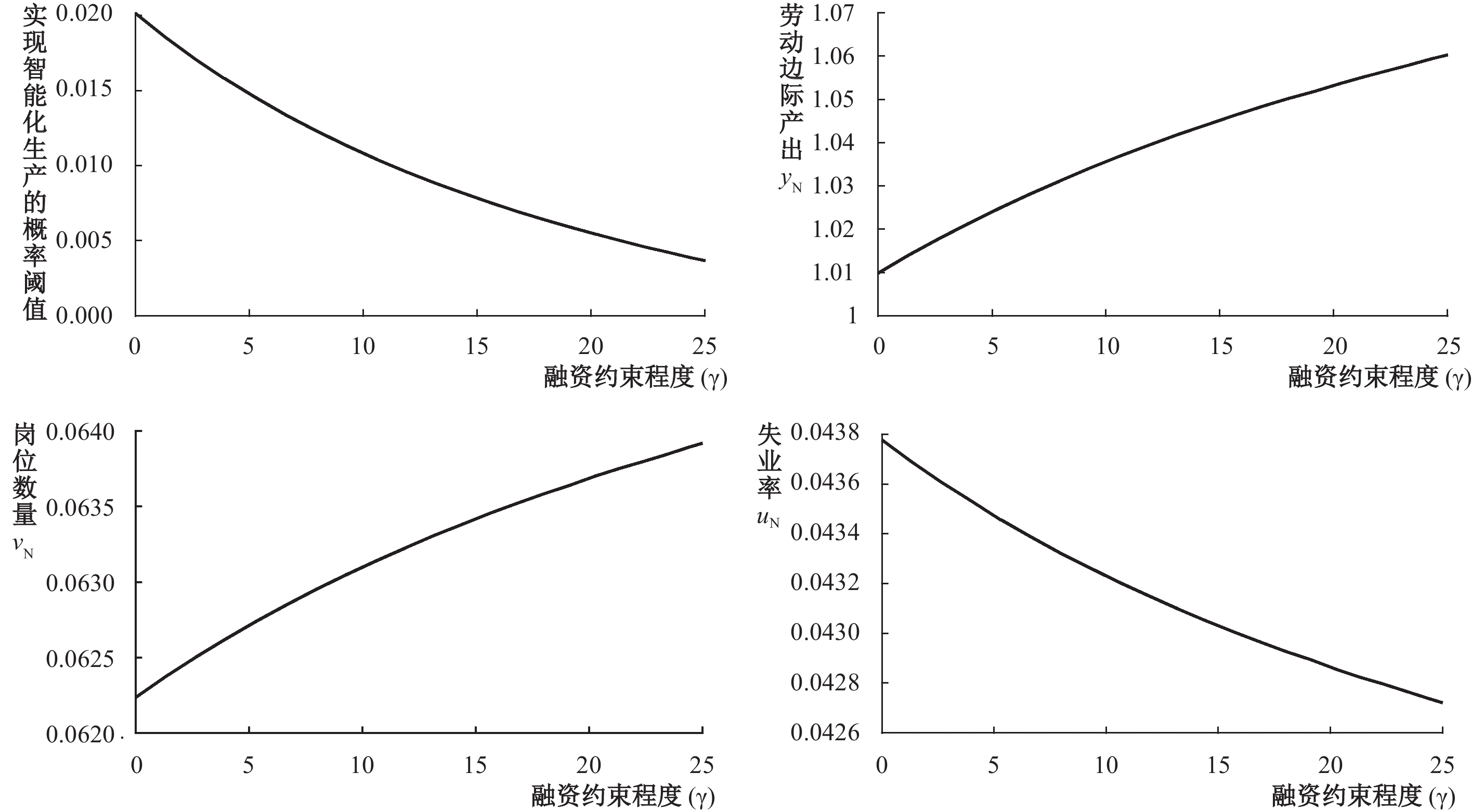图 5 机器替代效应和岗位创造效应的机制检验

4. 敏感性分析

① 我们没有按常规任务和非常规任务、简单任务和复杂任务来划分生产任务能否被人工智能替代。原因在于根据Frey和Osborne（2017），机器学习、数据挖掘、机器视觉、大数据等领域人工智能技术的发展使得更多非常规任务与复杂任务被智能资本替代，而人工智能技术在感知和操控类任务、创造性智能任务和社交智能任务上仍有所欠缺，需要上述技能的生产任务被机器替代的可能性较小。因此，我们按照实现人工智能技术替代的难易程度由低到高对不同生产任务进行排序。

② 虽然该任务与任务 ${i} \in\left(\alpha, 1\right)$ 均使用劳动力生产，但两者含义不同。前者表示该任务可以通过人工智能技术研发实现机器替代，但出于经济考虑，企业不进行研发，而是使用劳动力生产。后者表示该任务不具备人工智能机器替代劳动生产的可能性，仅能使用劳动力生产。由于两类岗位均使用劳动力生产，为了论证过程更简洁，我们在模型构建过程中没有区分两类岗位，而是均使用 $N$ 表示其岗位状态。

③ 由于篇幅所限，文章的证明过程都省略，读者若是感兴趣可向作者索取。

④ 由于篇幅所限，证明过程省略，读者若是感兴趣可向作者索取。

⑤ 由于篇幅所限，证明过程省略，读者若是感兴趣可向作者索取。

⑥ 使用可被人工智能替代的生产任务占比的不同值仅会导致定量结果差异，并不会导致不同的结论。

⑦ 任务 $i \in \left( {{\alpha _c},1} \right)$ 均由劳动力生产，稳态特征均相同，因此我们省略了任务（0.3, 1）的区间。

⑧ 由于篇幅所限，分析表格省略，读者若是感兴趣可向作者索取。

  胡晟明, 王林辉, 朱利莹. 工业机器人应用存在人力资本提升效应吗?[J]. 财经研究, 2021(6): 61–75.  贾俊生, 伦晓波, 林树. 金融发展、微观企业创新产出与经济增长——基于上市公司专利视角的实证分析[J]. 金融研究, 2017(1): 99–113.  解维敏, 方红星. 金融发展、融资约束与企业研发投入[J]. 金融研究, 2011(5): 171–183.  鞠晓生, 卢荻, 虞义华. 融资约束、营运资本管理与企业创新可持续性[J]. 经济研究, 2013(1): 4–16.  孔高文, 刘莎莎, 孔东民. 机器人与就业——基于行业与地区异质性的探索性分析[J]. 中国工业经济, 2020(8): 80–98. DOI:10.3969/j.issn.1006-480X.2020.08.006  李磊, 王小霞, 包群. 机器人的就业效应: 机制与中国经验[J]. 管理世界, 2021(9): 104–118.  孙早, 侯玉琳. 工业智能化如何重塑劳动力就业结构[J]. 中国工业经济, 2019(5): 61–79.  王林辉, 胡晟明, 董直庆. 人工智能技术会诱致劳动收入不平等吗——模型推演与分类评估[J]. 中国工业经济, 2020(4): 97–115.  王永钦, 董雯. 机器人的兴起如何影响中国劳动力市场? ——来自制造业上市公司的证据[J]. 经济研究, 2020(10): 159–175.  闫雪凌, 朱博楷, 马超. 工业机器人使用与制造业就业: 来自中国的证据[J]. 统计研究, 2020(1): 74–87.  杨飞, 范从来. 产业智能化是否有利于中国益贫式发展?[J]. 经济研究, 2020(5): 150–165.  杨光, 侯钰. 工业机器人的使用、技术升级与经济增长[J]. 中国工业经济, 2020(10): 138–156. DOI:10.3969/j.issn.1006-480X.2020.10.008  张晓娣. 公共部门就业对宏观经济稳定的影响——基于搜索匹配模型的DSGE模拟与预测[J]. 中国工业经济, 2016(4): 39–56.  郑江淮, 冉征. 智能制造技术创新的产业结构与经济增长效应——基于两部门模型的实证分析[J]. 中国人民大学学报, 2021(6): 86–101. DOI:10.3969/j.issn.1000-5420.2021.06.012  庄毓敏, 储青青, 马勇. 金融发展、企业创新与经济增长[J]. 金融研究, 2020(4): 11–30.  Acemoglu D, Restrepo P. The race between man and machine: Implications of technology for growth, factor shares, and employment[J]. American Economic Review, 2018, 108(6): 1488–1542. DOI:10.1257/aer.20160696  Acemoglu D, Restrepo P. Robots and jobs: Evidence from US labor markets[J]. Journal of Political Economy, 2020, 128(6): 2188–2244. DOI:10.1086/705716  Acemoglu D, Restrepo P. Demographics and automation[J]. The Review of Economic Studies, 2022, 89(1): 1–44. DOI:10.1093/restud/rdab031  Aghion P, Bergeaud A, Cette G, et al. Coase lecture: The inverted-U relationship between credit access and productivity growth[J]. Economica, 2019, 86(341): 1–31. DOI:10.1111/ecca.12297  Aghion P, Howitt P, Mayer-Foulkes D. The effect of financial development on convergence: Theory and evidence[J]. The Quarterly Journal of Economics, 2005, 120(1): 173–222.  Arntz M, Gregory T, Zierahn U. Revisiting the risk of automation[J]. Economics Letters, 2017, 159: 157–160. DOI:10.1016/j.econlet.2017.07.001  Battisti M, Felbermayr G, Peri G, et al. Immigration, search and redistribution: A quantitative assessment of native welfare[J]. Journal of the European Economic Association, 2018, 16(4): 1137–1188. DOI:10.1093/jeea/jvx035  Beck T, Levine R. Industry growth and capital allocation: Does having a market- or bank-based system matter?[J]. Journal of Financial Economics, 2002, 64(2): 147–180. DOI:10.1016/S0304-405X(02)00074-0  Brown J R, Martinsson G, Petersen B C. Do financing constraints matter for R&D?[J]. European Economic Review, 2012, 56(8): 1512–1529. DOI:10.1016/j.euroecorev.2012.07.007  Cortes G M, Jaimovich N, Siu H E. Disappearing routine jobs: Who, how, and why?[J]. Journal of Monetary Economics, 2017, 91: 69–87. DOI:10.1016/j.jmoneco.2017.09.006  Frey C B, Osborne M A. The future of employment: How susceptible are jobs to computerisation?[J]. Technological Forecasting and Social Change, 2017, 114: 254–280. DOI:10.1016/j.techfore.2016.08.019  Hémous D, Olsen M. The rise of the machines: Automation, horizontal innovation, and income inequality[J]. American Economic Journal: Macroeconomics, 2022, 14(1): 179–223. DOI:10.1257/mac.20160164  Lankisch C, Prettner K, Prskawetz A. How can robots affect wage inequality?[J]. Economic Modelling, 2019, 81: 161–169. DOI:10.1016/j.econmod.2018.12.015  Liu Y. Labor market matching and unemployment in urban China[J]. China Economic Review, 2013, 24: 108–128. DOI:10.1016/j.chieco.2012.10.006  Lu C H. The impact of artificial intelligence on economic growth and welfare[J]. Journal of Macroeconomics, 2021, 69: 103342. DOI:10.1016/j.jmacro.2021.103342  Ma H, Qiao X, Xu Y. Job creation and job destruction in China during 1998-2007[J]. Journal of Comparative Economics, 2015, 43(4): 1085–1100. DOI:10.1016/j.jce.2015.04.001  Nedelkoska L, Quintini G. Automation, skills use and training[R]. OECD Social, Employment and Migration Working Papers No.202, 2018.  Oberfield E, Raval D. Micro data and macro technology[J]. Econometrica, 2021, 89(2): 703–732. DOI:10.3982/ECTA12807  Prettner K, Strulik H. Innovation, automation, and inequality: Policy challenges in the race against the machine[J]. Journal of Monetary Economics, 2020, 116: 249–265. DOI:10.1016/j.jmoneco.2019.10.012